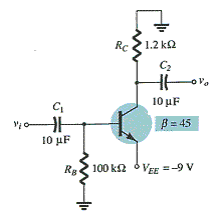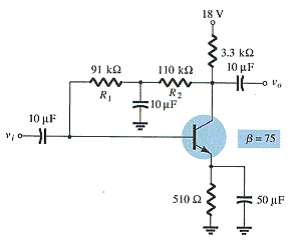# Practice Quiz in DC Biasing – BJT Part 4

(Last Updated On: February 3, 2020)

This is the Online Practice Quiz in DC Biasing – BJTs Part 4 from the book, Electronic Devices and Circuit Theory 10th Edition by Robert L. Boylestad. If you are looking for a reviewer in Electronics Engineering this will definitely help. I can assure you that this will be a great help in reviewing the book in preparation for your Board Exam. Make sure to familiarize each and every questions to increase the chance of passing the ECE Board Exam.

### Continue Part IV of the Online Practice Quiz

#### Quiz in DC Biasing – BJTs

Question 31. Which of the following is (are) related to an emitter-follower configuration?

A. The input and output signals are in phase.

B. The voltage gain is slightly less than 1.

C. Output is drawn from the emitter terminal.

D. All of these

Question 32. Calculate VCE.A. –4.52 V

B. 4.52 V

C. –9 V

D. 9 V

Question 33. Calculate Rsat if VCE = 0.3 V.A. 49.2 Ω

B. 49.2 kΩ

C. 49.2 mΩ

D. 49.2 MΩ

Question 34. Calculate ICsat.A. 35.29 mA

B. 5.45 mA

C. 1.86 mA

D. 4.72 mA

Question 35. Which of the following currents is nearly equal to each other?

A. IB and IC

B. IE and IC

C. IB and IE

D. IB, IC, and IE

Question 36. _____ should be considered in the analysis or design of any electronic amplifiers.

A. dc

B. ac

C. dc and ac

D. None of these

Question 37. A significant increase in leakage current due to increase in temperature creates _____between IB curves.

A. smaller spacing

B. larger spacing

C. the same space as at lower temperature

D. None of these

Question 38. In a fixed-bias circuit with a fixed supply voltage VCC’, the selection of a _____ resistor sets the level of _____ current for the operating point.

A. collector, base

B. base, base

C. collector, collector

D. None of these

Question 39. In a collector feedback bias circuit, the current through the collector resistor is _____ and the collector current is _____.

A. IC’ IC

B. IB + IC’ IC

C. IB’, IC

D. None of these

Question 40. In a fixed-bias circuit, the slope of the dc load line is controlled by _____.

A. RB

B. RC

C. VCC

D. IB

### More Practice Quiz in DC Biasing - BJTs

Practice Quiz Part 1

Practice Quiz Part 2

Practice Quiz Part 3

Practice Quiz Part 4

Practice Quiz Part 5

Practice Quiz Part 6

### See: Complete List of Practice Quizzes

Note: After taking this particular quiz, you can proceed to check all the topics.

Please do Subscribe on YouTube!

P inoyBIX educates thousands of reviewers and students a day in preparation for their board examinations. Also provides professionals with materials for their lectures and practice exams. Help me go forward with the same spirit.

“Will you subscribe today via YOUTUBE?”

Subscribe